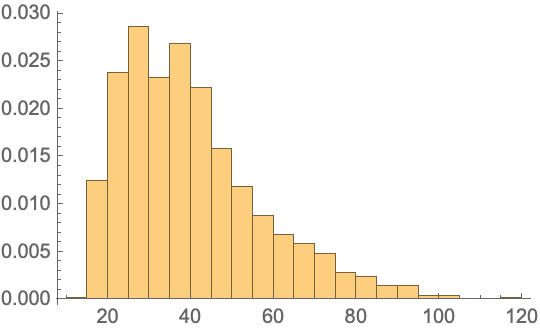#Function Repository Resource:

# CUSUMMaxRandomnessTest

Conduct a cumulative sum-based randomness test that creates a test statistic from the maximum value that a cumulative sums random walk achieves

Contributed by: Emmy/Noah Blumenthal
noahb320@gmail.com
emmyb320@bu.edu
 ResourceFunction["CUSUMMaxRandomnessTest"][sequence] conducts a cumulative sum-based randomness test on sequence. ResourceFunction["CUSUMMaxRandomnessTest"][sequence,"properties"] conducts a cumulative sum-based randomness test on sequence and returns the associated property.

## Details and Options

CUSUMMaxRandomnessTest creates a test statistic from the maximum value that a cumulative sums random walk achieves and returns the associated p-value.
Properties include:
 "TestStatistic" returns the test statistic "PValue" returns the p-value associated with the test
The test works only for sequences of zeros and ones.
Results are generally invalid for sequence lengths short than 100.
The test returns a two-tailed p-value of the distribution.

## Examples

### Basic Examples

Generate a sequence of random integers and apply a cumulative sums test:

 In:=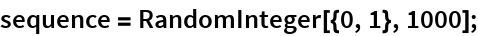Visualize the sequence:

 In:=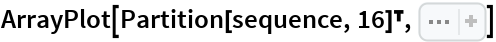Out=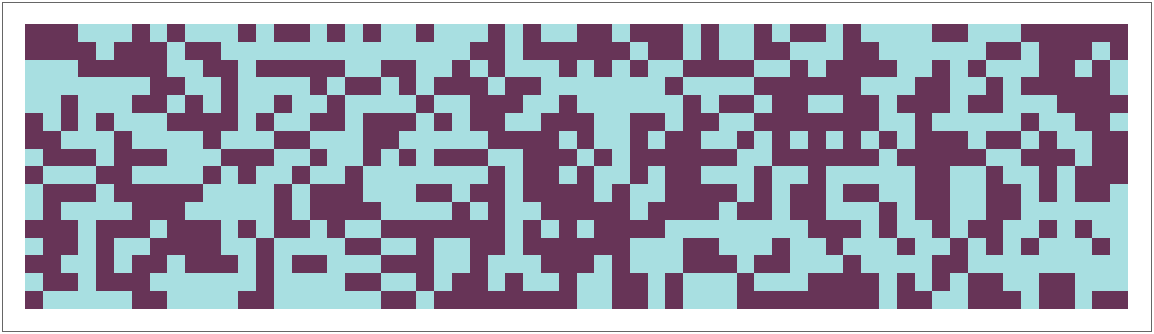In:=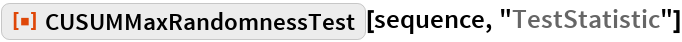Out=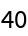In:=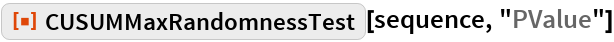Out=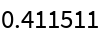### Applications

Reject a non-random sequence:

 In:=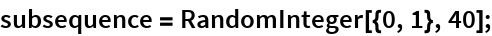In:=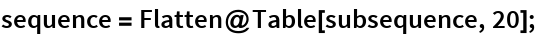In:=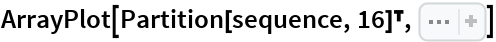Out=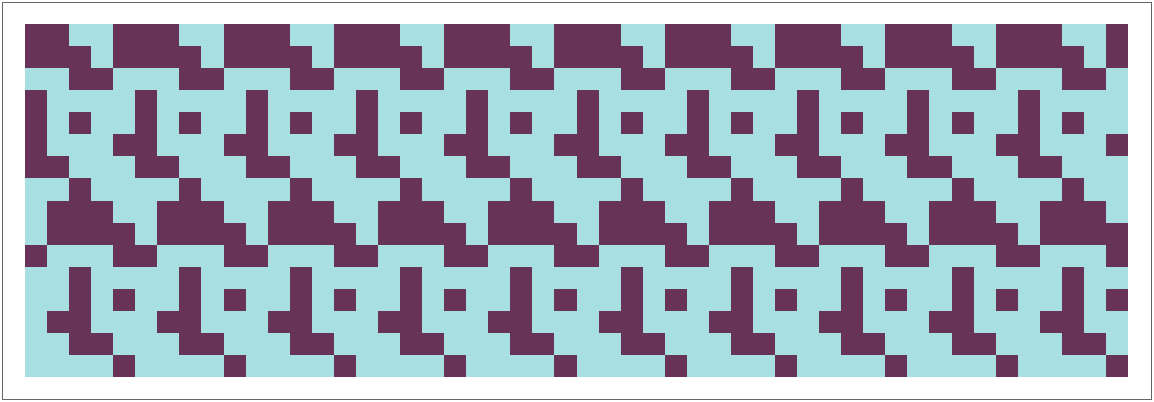In:=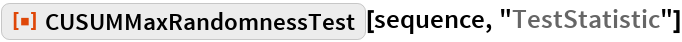Out=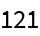In:=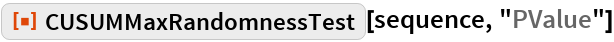Out=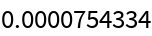Test the randomness of rule 30:

 In:=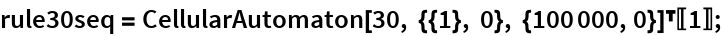In:=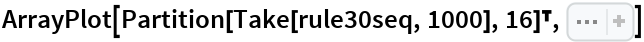Out=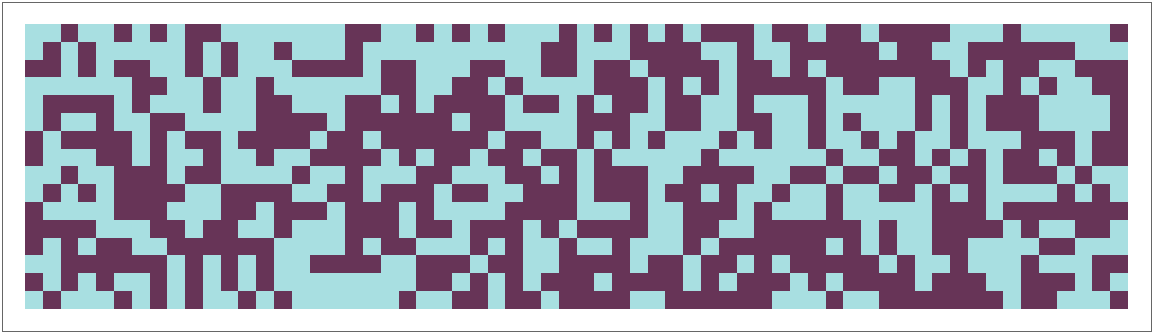In:=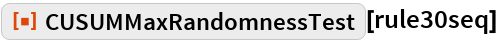Out=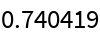### Possible Issues

CUSUMMaxRandomnessTest requires sequences of length 100 or more:

 In:=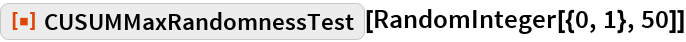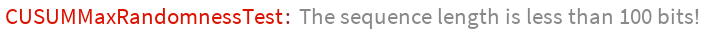### Neat Examples

Visualize the sampling distribution of the test statistic:

 In:=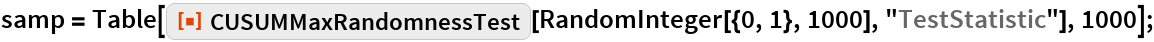In:=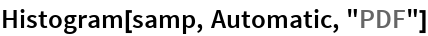Out=